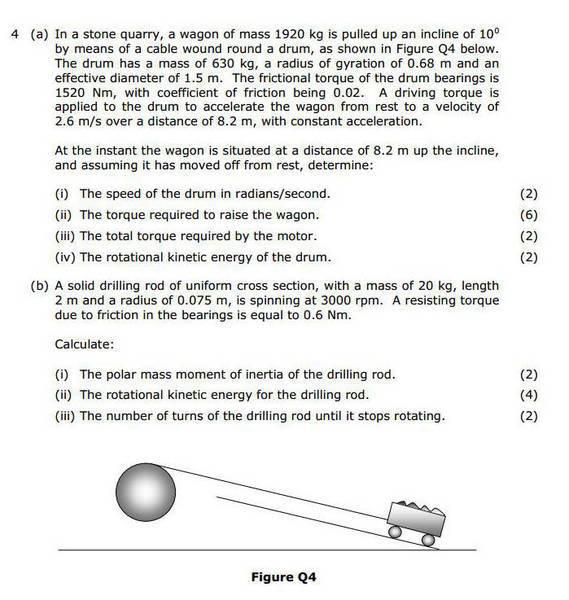# Polar moment of inertia in a rod?

• smr101

#### smr101

How is the polar moment of inertia in a rod calculated?

Thanks.

No idea what it is. This link something ?

Yeah, I've had a look at that. Formula doesn't quite make sense to me. I know what moment of inertia is but the 'polar' bit has thrown me off. Thanks anyway.

Since there is no context whatsoever in the original posting, it's difficult to assist any further here.

Since there is no context whatsoever in the original posting, it's difficult to assist any further here.

Here's the problem., 4. (b) (i) is the one I'm looking at.Well, at least now I can understand your confusion. However, it doesn't literally ask for the polar moment of inertia (a.k.a area moment of inertia), but for the polar mass moment of inertia. I see that used for the rotational moment of inertia, so with the context of b (ii) and b(iii) that seems the most logical choice.

Well, at least now I can understand your confusion. However, it doesn't literally ask for the polar moment of inertia (a.k.a area moment of inertia), but for the polar mass moment of inertia. I see that used for the rotational moment of inertia, so with the context of b (ii) and b(iii) that seems the most logical choice.

What is the formula for rotational moment of inertia?

Can't imagine you haven't seen it before ! What did you use for 4a ?

## I=\int dI = {\displaystyle \int_0^M r^2 \; dm}##

Can't imagine you haven't seen it before ! What did you use for 4a ?

## I=\int dI = {\displaystyle \int_0^M r^2 \; dm}##

I'm on 4. (a) (ii) currently. Where should it be used in (a)?

Ok, thanks, I have seen it but haven't used it previously.

Can't imagine you haven't seen it before ! What did you use for 4a ?

## I=\int dI = {\displaystyle \int_0^M r^2 \; dm}##

I've found this... http://hyperphysics.phy-astr.gsu.edu/hbase/mi2.html#irod3

Looking at the bottom set of equations, so dm = (M/L) x dr...

So essentially, the equation is r^2 x (M/L) x dr...

Using these number that would be 0.075^2 x (20/2) x 0.15 x 0.075...

Is that correct?

Tempting indeed (*), but I think this time the rod is turning around its length axis. I.e. it's a solid cylinder !

And you will need a moment of inertia in 4a (ii) as well: you don't just have to accelerate the cart, but also the drum.
However, in 4a (ii) they give you a radius of gyration, so you use that to calculate the moment of inertia..

(*) well, not really. did you really think dr stands for diameter x radius (because I see a 0.15 x 0.075, and then a few dots, indicating justified doubt, I hope) ?

Ok, I am familiar with the equation for mass moment of inertia for a cylinder, which fits with this.

So are you saying the equation you gave me wouldn't work with this? I find it odd they say it's a rod and expect a cylinder equation to be used.

And yes I knew it wasn't the correct answer as I have the solutions and it didn't match...I see that dr is used in the integration equation now.

So what is the formula?
dm = elemental mass =
(M/L) x dr so the whole equation is r^2 x (M/L) x dr
And then?

It's a drilling rod. Look here